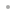Global OptimizationGlobal OptimizationFeaturesExamplesPackage FunctionsDownload Product ManualBuy OnlineRequest a Free Trial# Package Functions

Ten functions are provided in the package:

• GlobalSearch is a hill-climbing algorithm for nonlinear functions with analytic equality and inequality constraints. It is designed to be robust to local minima and to solve problems with hundreds of variables. No derivatives are required, and the user-defined objective function can even be nondifferentiable. Multiple starts allow the user to find multiple minima if they exist. This function is ideal for nonlinear regression, engineering design, model estimation, financial analysis, and other applications. Neither bounds nor a close initial guess are needed.
• GlobalPenaltyFn is a hill-climbing algorithm for nonlinear functions with nonanalytic equality and inequality constraints. It is designed to be robust to local minima and to solve problems with hundreds of variables. No derivatives are required, and the user-defined objective function can even be nondifferentiable. Multiple starts allow the user to find multiple minima if they exist. Neither bounds nor a close initial guess are needed.
• IntervalMin solves problems using interval methods. It is designed to be robust to local minima, and the problems can have inequality constraints. No derivatives are required, and the user-defined objective function can even be nondifferentiable. This function is ideal for nonlinear regression, engineering design, model estimation, financial analysis, and other applications.
• NLRegression solves nonlinear regression problems. A sensitivity analysis of parameter values around the solution point is provided. Confidence intervals are computed. Both L1 and L2 norms can be used. Constrained regression problems can also be solved.
• MaxLikelihood solves maximum likelihood estimation problems. Summary statistics are provided. Problems can be constrained to obtain better solutions. A library of speed-optimized common univariate functions is provided.
• InterchangeMethodMin is a function for 0-1 integer problems with a linear or nonlinear objective function. It can solve routing, traveling salesman, minimal spanning tree, and other discrete network problems even when the objective function is nonlinear.
• TabuSearchMin is a function for 0-1 integer problems with a linear or nonlinear objective function and is related to the interchange method approach described previously. The "tabu" feature increases efficiency on complex problems. It can solve routing, traveling salesman, minimal spanning tree, and other discrete network problems even when the objective function is nonlinear.
• GlobalMinima solves smaller constrained or unconstrained global nonlinear models. This algorithm is based on the identification of feasible points that define the solution set at each iteration. As lower points are found during the grid refinement process, points far from the current optimum are pruned from the solution set. As a result, multiple minima, if they exist, can be found in a single run. The algorithm can also identify optimal regions rather than only single points. These optimal regions might represent the bounds on feasible management strategies that achieve an equivalent result, or they might depict confidence limits for a parameter estimation problem.
• MaxAllocation is designed for allocation problems such as arise in investment, where a fixed amount of money is to be allocated across a series of investment options. Such problems have a single equality constraint and a positivity restriction on all variables. The path-following algorithm used is able to solve this type of problem with high efficiency, and can handle problems over a thousand variables. This function is ideal for quadratic programming, investment allocation, and hedge-fund creation applications.